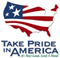# The National Flood-Frequency Program—Methods for Estimating Flood Magnitude and Frequency in Rural Areas in Hawaii, Island of Oahu, 2000

#### U.S. Geological Survey Fact Sheet 004-00 October, 2000

This report is available in pdf 498KB.

## Introduction

Estimates of the magnitude and frequency of flood-peak discharges and flood hydrographs are used for a variety of purposes, such as for the design of bridges, culverts, and flood-control structures; and for the management and regulation of flood plains. To provide simple methods of estimating flood-peak discharges, the U.S. Geological Survey (USGS) has developed and published regression equations for every State, the Commonwealth of Puerto Rico, American Samoa and a number of metropolitan areas in the United States. In 1993, the USGS, in cooperation with the Federal Emergency Management Agency and the Federal Highway Administration, compiled all current USGS statewide and metropolitan area regression equations into a computer program, titled “The National Flood-Frequency (NFF) Program” (Jennings and others, 1994).

Since 1993, new or updated regression equations have been developed by the USGS for various areas of the Nation. These new equations have been incorporated into an updated version of the NFF Program.

This fact sheet describes the application of the updated NFF Program to streams that drain rural areas in Hawaii, island of Oahu. Information on obtaining the NFF software and fact sheets for other areas of the Nation is provided at the end of this Fact Sheet.

## Overview

Wong (1994) developed regression equations for estimating peak discharges (QT), in cubic feet per second, having recurrence intervals (T) ranging from 2 to 100 years, for the island of Oahu, Hawaii. Separate sets of equations were developed for each of three hydrologic regions: (1) leeward, (2) windward, and (3) north (fig. 1).

Recurrence interval is the reciprocal of the annual exceedance probability and represents the average number of years between exceedances. For example, a flood-peak discharge with an exceedance probability of 0.01 has a recurrence interval of 100 years. This does not imply that the 100-year flood will be equaled or exceeded each 100 years, but that it will be equaled or exceeded on the average once every 100 years.

The regression equations shown in table 1 were developed from peak-discharge records available through 1988 and basin and climatic characteristics for 79 continuous and crest-stage stations on Oahu. These peak-discharge records and characteristics were presented for the 79 stations in tabular form by Wong (1994). The mean record length for the stations was 30 years, and ranged from 11 to 72 years. Generalized-least-squares regression techniques were used to develop the equations (Tasker, 1982).

The regression equations are not applicable to sites subject to diversion, regulation, or some proportion of urbanization. For Oahu, urbanization is considered substantial when the urban cover, defined below, is greater than 36 percent (Wong, 1994).

## Procedure

The equations in table 1 are based on the inch-pound system of units, but the NFF Program will accept and report either the inch-pound or metric system of units. The explanatory watershed and climatic variables used in the regression equations are as follow: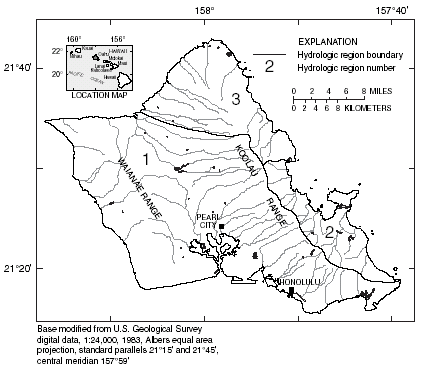Figure 1. Hydrologic regions for the Island of Oahu, Hawaii.

 Regression equation Bias correction Average factor standard error of prediction in percent Table 1. Flood-peak discharge regression equations and associated statistics for streams that drain rural areas in Hawaii, island of Oahu (modified from Wong, 1994) [QT, flood-peak discharge, in cubic feet per second, for recurrence interval T, 2 to 100 years; DA, drainage area, in square miles; P, median annual precipitation, in inches; P224, 2-year, 24-hour precipitation intensity, in inches] Q2 = 3.26DA0.634P1.08 1.115 43 4.2 Q5 = 25.8DA0.642P0.773 1.069 40 5.8 Q10 = 73.5DA0.646P0.621 1.052 39 8.2 Q25 = 217DA0.646P0.464 1.040 38 11.4 Q50 = 425DA0.645P0.368 1.037 38 13.7 Q100 = 758DA0.643P0.286 1.040 39 15.8 Q2 = 525DA0.704 1.165 62 2.5 Q5 = 1,140DA0.748 1.138 58 3.9 Q10 = 1,700DA0.763 1.129 54 5.7 Q25 = 2,580DA0.773 1.124 52 8.6 Q50 = 3,360DA0.776 1.125 51 11.0 Q100 = 4,250DA0.777 1.133 50 13.6 Q2 = 0.00356DA0.870P2245.85 1.036 45 3.6 Q5 = 0.151DA0.836P2244.30 1.000 34 8.3 Q10 = 1.76DA0.805P2243.24 1.000 34 10.2 Q25 = 24.8DA0.777P2242.10 1.000 38 10.7 Q50 = 125DA0.765P2241.39 1.000 43 10.5 Q100 = 500DA0.758P2240.792 1.011 48 10.1

Drainage area (DA), in square miles, is the total area that contributes runoff upstream of the stream site of interest. Drainage area is determined from 1:24,000-scale USGS topographic maps by planimeter.

Median annual precipitation (P), in inches, is the average median precipitation for the drainage basin and is determined by the grid-sampling method from an equal-precipitation map developed by the Division of Water and Land Development (1982).

2-year, 24-hour precipitation (P224), in inches, is the average of the 2-year recur- rence interval, 24-hour duration storm determined for the drainage basin from a map by Giambelluca and others (1984) by use of the grid-sampling method.

Urban cover (UC), in percent, is not included as an explanatory variable in the regression equations, but it should be measured because the equations do not apply for basins with greater than the percentages of urban area shown in table 2. UC can be determined by measuring urban areas within the basin of interest, shown in pink on 1:24,000-scale USGS topographic maps, and dividing the sum of the areas by the drainage area of the basin, and multiplying the result by 100.

 Hydrologic study region Drainage area Median annual 2-year, in square miles rainfall, 24-hour in inches rainfall intensity, in inches Table 2. Range of explanatory variables for which regression equations are applicable (modified from Wong, 1994) Region 1 (Leeward) 0.60–45.7 29–239 4.72–8.78 0–32 Region 2 (Windward) 0.03–5.34 52–146 5.62–9.10 0–36 Region 3 (North) 1.11–13.5 66–197 5.21–9.04 0

A Bias Correction Factor (BCF) is used to correct the retransformation bias introduced by the logarithmic transformation of the dataset used to develop the regression equations. Use of the BCF in the regression equation yields the mean value of the estimated peak discharge, whereas not using the BCF yields the less conservative median value of the estimated peak discharge. Users of the NFF Program do not need to enter the BCF values because they have been incorporated into the equations.

The regression equations, the bias correction factors, the average standard errors of prediction, and the equivalent years of record are shown in table 1. The average standard error of prediction is a measure of the accuracy of a regression equation when used to estimate peak discharges for ungaged watersheds similar to those used to derive the regression equation. Errors in the QT estimates for about two-thirds of the ungaged sites will be within the given standard errors. Errors increase appreciably when any explanatory variable is near or beyond the range limits shown in table 2. The equivalent years of record is the number of years of stream- flow records needed to achieve the same accuracy as the regression equation.

## Improving Estimates With Gaged Data

Wong (1994) developed weighting techniques to improve estimates of peak discharge at gaged locations by combining the estimates derived from analysis of gage records with estimates derived from the regression equations. The weights of these two independent estimates are based on the length of the gage record (in years) and the equivalent years of record of the applicable regression equation. The weighted estimate of peak discharge is computed as: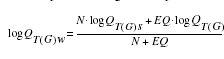QT(G)w is the weighted estimate of peak discharge, QT, for recurrence interval T at the gage location, QT(G)s is the estimate of QT derived from analysis of the systematic gage records, QT(G)r is the estimate of QT derived from application of the appropriate regression equation in table 1, N is the number of years in the gage record used to compute QT(G)s, and EQ is the equivalent years of record (table 1).

The accuracy of the weighted discharge estimate, in equivalent years of record, is equal to N + EQ. The NFF Program performs these computations.

## Ungaged Sites Near Gaged Sites on the Same Stream

Wong (1994) showed how the weighted estimate of peak discharge at a gaged site can be used to improve estimates of peak discharge for an ungaged site on the same stream that has a drainage that is between 50 and 150 percent of the drainage area of the gaged site. The regression estimate for the ungaged site is multiplied by an adjustment factor, which is computed as: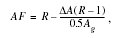where

 AF is the adjustment factor,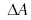is the absolute value of difference in drainage area between the gaged site (Ag) and the ungaged site (Au), |Ag -Au|, and R is the ratio of the weighted peak- discharge estimate to the regression estimate for the gaged site, QT(G)w/QT(G)r.

The equations are used without adjustment where the drainage area of the ungaged site is not within 0.5 to 1.5 times the drainage area of the gaged site.

—Prepared by Robert R. Mason, Jr., and Luis A. Fuste, of the U.S. Geological Survey; and Jeffrey N. King and Wilbert O. Thomas, Jr., of Michael Baker, Jr., Inc.

## References

Division of Water and Land Development, 1982, Median rainfall, State of Hawaii: Department of Land and Natural Resources, State of Hawaii, Circular C88, 44 p.

Giambelluca, T.W., and others, 1984, Rainfall frequency study for Oahu: Division of Water and Land Development, Department of Natural Resources, State of Hawaii, Report R–73, 34 p.

Jennings, M.E., Thomas, W.O., Jr., and Riggs, H.C., comps., 1994, Nationwide summary of U.S. Geological Survey regional regression equations for estimating magnitude and frequency of floods for ungaged sites, 1993: U.S. Geological Survey Water- Resources Investigations Report 94–4002, 196 p.

Tasker, G.D., 1982, Hydrologic regression with weighted least squares: Water Resources Research, v. 16, no. 6., p. 1107-1113.

Wong, M.F., 1994, Estimation of magnitude and frequency of floods for streams on the island of Oahu, Hawaii: U.S. Geological Survey Water- Resources Investigations Report 94– 4052, 37 p.

U.S. Geological Survey

Office of Surface Water

415 National Center

Reston, Virginia 20192

(703) 648-5301

USGS hydrologic analysis software is available for electronic retrieval through the World Wide Web (WWW) at: http://water.usgs.gov/software/ and through anonymous File Transfer Protocol (FTP) from water.usgs.gov (directory:/pub/software). The WWW page and FTP directory from which the National Flood-Frequency software and user documentation can be retrieved are http://water.usgs.gov/software/nff.html and /pub/software/surface_water/nff, respectively.

Additional earth science information is available from the USGS through the WWW at http://www.usgs.gov/ or by calling 1-888-ASK-USGS.

 AccessibilityFOIAPrivacyPolicies and Notices U.S. Department of the Interior, U.S. Geological Survey Persistent URL: Page Contact Information: Contact USGS Last modified: Tuesday, November 29 2016, 05:53:11 PM